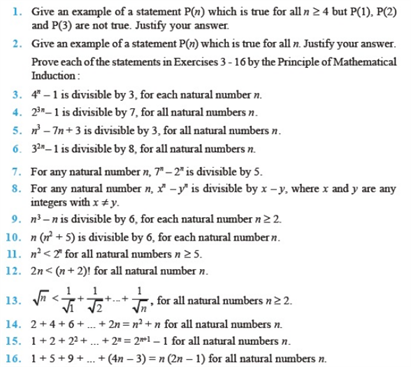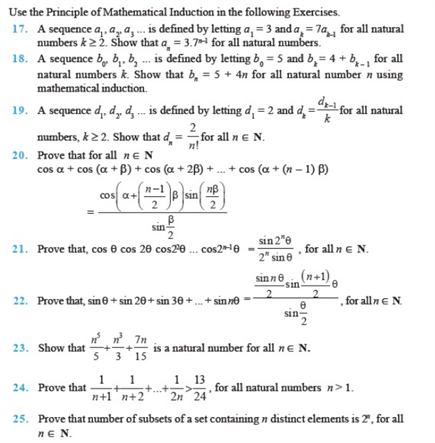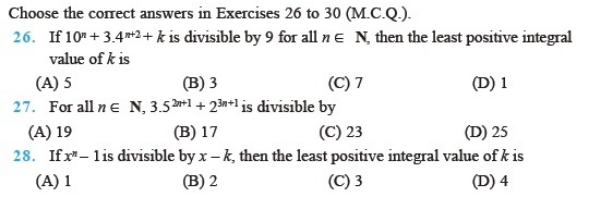# Chapter 4 - Principles of Mathematical Induction

Chapter 4 Mathematical Induction of class 11 includes problems or statements which involves mathematical relations. It is one of the important topics of class 11. We have given a few important questions of chapter 4 – Principles of Mathematical Induction in this page. Solving these problems will help you in scoring well in exams.

Also, check:

Mathematical Induction – Basics

## Important Questions on Principle of Mathematical Induction, Chapter 4 Class 11

1. Show that n(n+1)(2n +1) is divisible by 6 for al n belong to N(Use the principle of mathematical induction).

2. Prove by induction that the sum of the cubes of three consecutive natural numbers is divisible by 9.

3. State the first principle of mathematical induction.

4. Prove by using the principle of mathematical induction: – 2 + 5 + 8 + 11 + …+(3n – 1) = 1/2n(3n+1).

5. 1 + 3 + 32  +… + 3n-1  = (3n – 1) / 2, Use mathematical induction t prove LHS = RHS.

### NCERT Questions of Chapter 4 Class 11 – Principle of Mathematical Induction